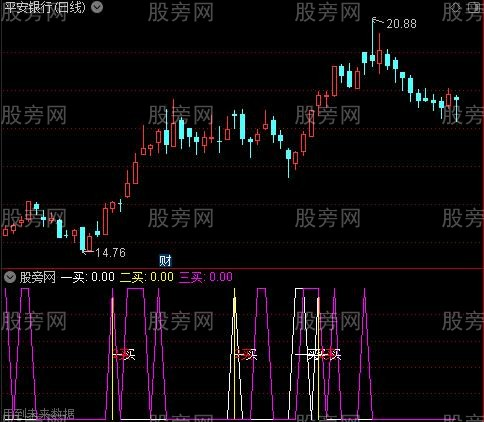# Stock Liao information

— Basic knowledge of stocks|Introduction to basics of stocks|Stock learning|Basic knowledge of stocks
Mobile access：m.liaochihuo.com

# Tongdaxin buy point index formula

Release Time:2021-07-09 Topic:Tongdaxin Volume and Price Angle Index Formula Reading：13 Navigation：Stock Liao information > Current Events > Tongdaxin buy point index formula

DIF:=EMA(CLOSE,12)-EMA(CLOSE,26);

DEA:=EMA(DIF,9);

MACD:=2*(DIF-DEA);

QZQ:=BARSLAST(REF(MACD,1)0);

QM:=LLVBARS(MACD,QZQ+20);

MQDZ:=IF(QM>QZQ,REF(MACD,QM),0);

XG1:=MACDMQDZ

AND CLOSE=LLV(CLOSE,QZQ+20) AND M(MA(C,10),1) AND MA(C,10)>REF(MA(C,21),1)

AND MA(C,21)>REF(MA(C,21),1) ;

Angle MA5:=ATAN((MA5/REF(MA5,1)-1)*100)*180/3.1416;

Angle MA7:=ATAN((MA7/REF(MA7,2)-1)*100)*180/3.1416;

Angle MA13:=ATAN((MA13/REF(MA13,4)-1)*100)*180/3.1416;

Angle MA20:=ATAN((MA20/REF(MA20,4)-1)*100)*180/3.1416 ;

Angle MA34:=ATAN((MA34/REF(MA34,3)-1)*100)*180/3.1416 ;

Angle MA55:=ATAN((MA55/REF(MA55,2)-1)*100)*180/3.1416 ;

Angle MA120:=ATAN((MA120/REF(MA120,3)-1)*100)*180/3.1416 ;

Angle 10 daily average volume: =ATAN((V10/REF(V10,2)-1)*100)*180/3.1416;

Angle 20 daily average volume: =ATAN((V20/REF(V20,2)-1)*100)*180/3.1416;

Angle 34 daily average volume: =ATAN((V34/REF(V34,2)-1)*100)*180/3.1416 ;

Angle 60 daily average amount: =ATAN((V60/REF(V60,3)-1)*100)*180/3.1416;

Angle 90 daily average volume: =ATAN((V90/REF(V90,3)-1)*100)*180/3.1416 ;

stock selection: =angle MA13>=20 AND angle MA20>=15 AND angle MA34 >=-10 AND MA(C,13)>MA(C,20) AND MA(C,20)>REF(MA(C,20),1);

Stock pick 2: =angle MA13>=45 AND angle MA20>=10 AND angle MA20=-10;

Stock pick 3:=angle MA13=MA13 AND MA13>=MA20*0.978;

Long 2:=(MA20>=MA34*0.98 AND angle MA34>=30) OR (MA20>=MA55*0.98 AND angle MA55>=30) OR (MA20>=MA120*0.978 AND angle MA120>=30) ;

Moving Average Long: = Long 1 AND Long 2 AND C>=MA20 AND O>=MA20;

Average amount angle 1:=Angle 10 daily average amount>=80 AND Angle 20 daily average amount>=80;

Average amount angle 2: = angle 20 daily average amount>=80 AND angle 34 average daily volume>=80;

Average amount angle 3:=Angle 10 daily average amount>=80 AND Angle 34 daily average amount>=80;

Average angle: = average angle 1 OR average angle 2 OR average angle 3;

Volume long 1:= V5>=V10*0.94 AND V10>=V20 AND V20>=V60*0.94 AND V60>=V90*0.93;

Quantity long 2:= V5>=V10*0.94 AND V10>=V20*0.94 AND average angle;

Volume Long: = Volume Long 1 OR Volume Long 2;

Daily limit 1: = ((C-REF(C,1))/REF(C,1))>=0.095;

Today's volume: = daily limit 1 OR V>=V10;

increase: = ((C-REF(C,1))/REF(C,1)>=0.023 OR (CO)/O)>=0.023;

Heap 1:=(V+REF(V,1)+REF(V,2))/3;

Pile volume 11:=(V+REF(V,1)+REF(V,2)+REF(V,3))/4;

Stack 2:=(REF(V,4)+REF(V,5)+REF(V,6)+REF(V,7)+REF(V,8))/5;

Stack amount 3:=(REF(V,9)+REF(V,5)+REF(V,6)+REF(V,7)+REF(V,8))/5;

Stack amount 4:=(REF(V,9)+REF(V,10)+REF(V,6)+REF(V,7)+REF(V,8))/5;

Heap amount: = Heap amount 1 / Heap amount 2>=1.9 OR Heap amount 1 / Heap amount 3>=1.9 OR Heap amount 1 / Heap amount 4>=1.9 OR Heap amount 11/ Heap amount 2>=1.9 OR Heap amount 11/heap volume 3>=1.9 OR heap volume 11/heap volume 4>=1.9;

Amount 0: = Amount AND increase AND C>=MA7 AND C>=MA20 AND C>O;

A:=COUNT(ZIG(3,5),20)=2;

D:=REF(C,B)>LLV(C,20);

E:=C>REF(C,B) ;

VAR2:=LLV(LOW,A);

VAR3:=HHV(HIGH,B);

Power line: =EMA((CLOSE-VAR2)/(VAR3-VAR2)*4,4);

Multi 1: =DIF>REF(DIF,1);

Multi2: =DEA>REF(DEA,1);

Lot 3: =MACD>REF(MACD,1);

Multi 4: =EVERY(DIF>REF(DIF,1),3);

Multi 5: =EVERY(DEA>REF(DEA,1),3);

Multi 6: =EVERY(MACD>REF(MACD,1),3);

Multi 7: =CROSS(DIF,DEA);

Lot 8:=COUNT(Lo 7,20);

Multi 9: =MACD>0;

Empty 1:=DIFO)));

One buy 2:=FILTER(CROSS(power line,0.5),20) OR FILTER(CROSS(power line,0.2),20);

One buy 1:=(COUNT((quantity long AND today quantity),20)>0 AND COUNT(L0 AND C>=MA(C,5) AND MA(C,5)>REF(MA(C,5) ,1))OR(V>REF(HHV(V,60)*0.96,1) AND COUNT(L0);

One buy: one buy 3 OR (one buy 2 OR one buy 1 OR((REF(XG1,1)=1 AND MACD>REF(MACD,1)) OR(COUNT(REF(XG1,1)=1 AND MACD >REF(MACD,1),20)>0 AND LLV(L,60)0))AND C>=MA(C,5));

QF:=LLVBARS(DIF,QZQ+20);

DQDZ:=IF(QF>QZQ,REF(DIF,QF),0);

XG11:=MACDMQDZ AND DIFDQDZ

AND CLOSE=LLV(CLOSE,QZQ+20) AND MQDZ=MA(C,5))OR(COUNT(one buy,20)>0 AND COUNT(C>LLV(L,60),5)>0 AND MA (C,5)>REF(MA(C,5),1) AND CROSS(MA(C,5),MA(C,10)) AND C>=MA(C,5)) STICK;

N1:=9;{Last Decline Days}

N2:=30;{increasing days}

N3:=56;{days of platform high point}

LL:=LLV(L,N1);

HH:=HHV(H,2*N1);

H0:=REF(HHV(H,N3),N1+N2);

Three buy 0: =HH=HHV(H,N2+N1+N3) AND LL>H0 AND LL/HH=0.97*H01 AND LL1/HH1=H02;Article Url:https://www.liaochihuo.com/info/604630.html

Label group:[Tongdaxin index formula

• ### Frequent low-level errors in the application documents. Intermediaries write "typos" or be punished (with the latest IPO queue list)

Abstract The application documents frequently show lowlevel errors and the intermediary agency writes typos or is punished with the latest IPO queue l...

• ### ipo ranking latest ranking, ipo queuing

Content navigationWhere can I find the ranking of the Chinese ashare ipo business of accounting firms? The latest ranking of domestic securities com...

• ### The latest list of ipo queues in 2021 and 2021 will be listed on the science and technology board

This article comes from the official WeChat account of the forwardlooking IPO WeChat Upcoming meeting Meeting today Shanghai Visebo Environmental Prot...

• ### (Latest) List of companies queuing for listing in various industries in Hong Kong IPO! _ Wind

Original title latest Hong Kong IPO list of listed companies in various industries Source Daxiang IPO ID daxiangipo Please indicate the source for ...

• ### List of bank IPO queues in 2020, 18 banks queued up for IPO

A few days ago, Shanghai Rural Commercial Bank updated its prospectus application draft and joined the team of Rural Commercial Banks impact on Ashar...

• ### Shanghai Stock Exchange's ipo information query zone, queuing for listing on the ChiNext

As of December 13, 2018, the China Securities Regulatory Commission has accepted 278 companies that initiated the issuance of depository receipts, of ...

• ### What does it mean for ipo to terminate review, the latest ipo listing queue list

Content navigationThe IPO terminates the review. What is the essential difference between the termination of the review and the suspension of the re...

• ### Another bank with assets of 1700 billion will be held for IPO (with the latest IPO queue list)-Stock Channel-Hexun.com

Since 2019, the pace of banks rushing to Ashare IPOs has accelerated significantly. The latest announcement by the China Securities Regulatory Commis...